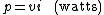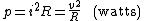# Electric power measurement

Also found in: Dictionary, Thesaurus, Medical.

## Electric power measurement

The measurement of the time rate at which electrical energy is being transmitted or dissipated in an electrical system. The potential difference in volts between two points is equal to the energy per unit charge (in joules/coulomb) which is required to move electric charge between the points. Since the electric current measures the charge per unit time (in coulombs/second), the electric power p is given by the product of the current i and the voltage &ugr; (in joules/second = watts), as in Eq. (1). (1)See E lectric current ; E lectrical units and standards ; P ower .

Alternate forms of the basic definition can be obtained by using Ohm's law, which states that the voltage across a pure resistance is proportional to the current through the element. This results in Eq. (2),

(2)where R is the resistance of the element and i and &ugr; are the current through and voltage across the resistive element. Other commonly used units for electric power are milliwatts (1 mW = 10-3 W), kilowatts (1 kW = 103 W), megawatts (1 MW = 106 W), and, in electromechanical systems, horsepower (1 hp = 746 W). See Ohm's law

These fundamental expressions yield the instantaneous power as a function of time. In the dc case where &ugr; and i are each constant, the instantaneous power is also constant. In all other cases where &ugr; or i or both are time-varying, the instantaneous power is also time-varying. When the voltage and current are periodic with the same fundamental frequency, the instantaneous power is also periodic with twice the fundamental frequency. In this case a much more significant quantity is the average power, since in most cases the electric power is converted to some other form such as heat or mechanical power and the rapid fluctuations of the power are smoothed by the thermal or mechanical inertia of the output system.

The measurement of power in a dc circuit can be carried out by simultaneous measurements of voltage and current by using standard types of dc voltmeters and ammeters. The product of the readings typically gives a sufficiently accurate measure of dc power. If great accuracy is required, corrections for the power used by the instruments should be made. In ac circuits the phase difference between the voltage and current precludes use of the voltmeter-ammeter method unless the load is known to be purely resistive. When this method is applicable, the instrument readings lead directly to average power since ac voltmeters and ammeters are always calibrated in rms values. See Ammeter, Voltmeter

In power-frequency circuits the most common instrument for power measurement is the moving-coil, dynamometer wattmeter. This instrument can measure dc or ac power by carrying out the required multiplication and averaging on a continuous analog basis. The instrument has four terminals, two for current and two for voltage, and reads the average power directly. It can be built with frequency response up to about 1 kHz. See Wattmeter

Electronic wattmeters are available which give a digital indication of average power. Their primary advantage, in addition to minimizing errors in reading the instrument, is that the frequency range can be greatly extended, up to 100 kHz or more, with good accuracy.

Measurement of the total power in a polyphase system is accomplished by combinations of single-phase wattmeters or by special polyphase wattmeters which are integrated combinations of single-phase wattmeter elements. A general theorem called Blondel's theorem asserts that the total power supplied to a load over N wires can be measured by using N - 1 wattmeters. The theorem states that the total power in an N-wire system can be measured by taking the sum of the readings of N wattmeters so arranged that each wire contains the current coil of one wattmeter. One voltage terminal of each wattmeter is connected to the same wire as its current coil, and the second voltage terminal is connected to a common point in the circuit. If this common point is one of the N wires, one wattmeter will read zero and can be omitted.

At frequencies significantly above power frequencies, dynamometer wattmeters become inaccurate and cannot be used. The newer digital wattmeters have usable ranges well above audio frequencies and make accurate audio-frequency power measurements quite feasible. Generally, however, power measurements at higher frequencies are based on indirect methods.

For frequencies up to a few hundred megahertz, the voltage across a standard resistance load can be measured and the power calculated from V2/R. Instruments which combine the resistive load and voltmeter are called absorption power meters.

A diode may be used as a detector for radio-frequency (rf) power measurement. This type of instrument is simple and easy to use but is less accurate than thermally based systems. See Diode

A thermocouple consists of two dissimilar metals joined at one end. When the joined end is heated and the other end is at a lower temperature, an electric current is produced (the thermoelectric or Seebeck effect). The current is proportional to the temperature difference between the two ends. For electric power measurements, the hot end is heated by a resistor supplied from the rf power source to be measured. Modern devices use thin-film techniques to make the thermocouple and resistor to ensure good thermal coupling. The output is a low-level dc signal as in diode detector systems. See Thermocouple

A bolometer is basically an electric bridge circuit in which one of the bridge arms contains a temperature-sensitive resistor. In principle, the temperature is detected by the bridge circuit. Bolometric bridges use either barreters or thermistors as the temperature-sensitive resistor. The thermistor, which has largely replaced the barreter since it is more rugged, is a semiconductor device with a negative temperature coefficient. For rf power measurements, the thermistor is fabricated as a small bead with very short lead wires so that essentially all the resistance is in the bead. See Bolometer, Thermistor

The most accurate high-frequency power measurement methods involve calorimetric techniques based on direct determination of the heat produced by the input power. The power to be measured is applied to the calorimeter, and the final equilibrium temperature rise is recorded. Then the input signal is removed, and dc power applied until the same equilibrium temperature is attained. The dc power is then the same as the signal power. Calorimeter methods are usually used in standards laboratories rather than in industrial applications.

McGraw-Hill Concise Encyclopedia of Engineering. © 2002 by The McGraw-Hill Companies, Inc.
Site: Follow: Share:
Open / Close